Next: 2-D Field Data Results Up: Particular Implementation of LSJIMP Previous: Velocity-Depth Ambiguity in the

## Solving the particular LSJIMP problem

Now that we have derived appropriate imaging and amplitude correction operators, we are ready to translate the general LSJIMP modeling equation () to my particular implementation. The primary image,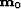, is mapped into data space primary events by NMO,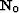. Similarly, a given pegleg image,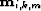, is mapped into data space by sequentially applying the differential geometric spreading correction (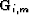), Snell resampling (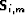), HEMNO (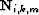), and finally, a reflection coefficient (). Let us rewrite equation () accordingly:(28)
We see that in equation (), the analog to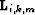in equation () is.

The data residual weight in equation (),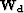, can often strongly influence the success of the inversion. Technically,carries a heavy burden: it must decorrelate and balance the residual. However, I have found that a simpler form fornontheless pays dividends. I set, which has the same dimension as a CMP gather, to zero where the data,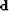, has an empty trace, and also above the onset of the seabed reflection.Next: 2-D Field Data Results Up: Particular Implementation of LSJIMP Previous: Velocity-Depth Ambiguity in the
Stanford Exploration Project
5/30/2004